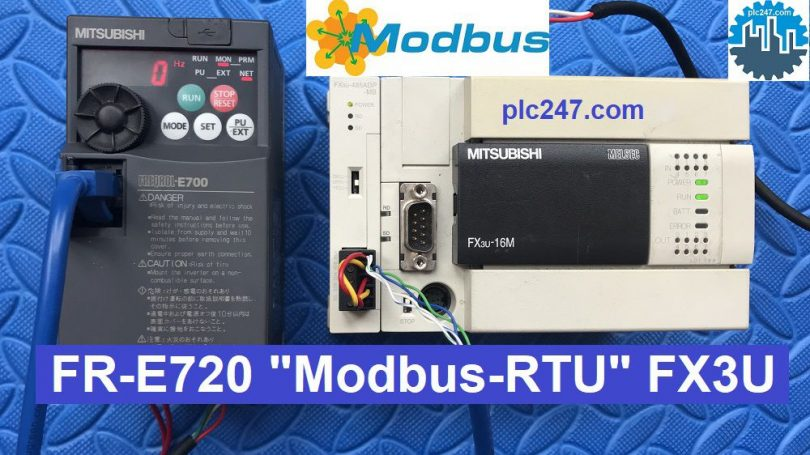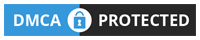# FX3U “Modbus RTU” FR-E700 Series Mitsubishi VFDWritten by

Hello everyone!
Today plc247.com would like to continue to guide you to control Mitsubishi E700 Series inverters with Modbus RTU standard by Mitsubishi’s own FX3U PLC (combined with FX3U-485ADP)

FR E700 Series has FX3U native communication mode support, however it does not support as many stations as Modbus RTU

### Communication Configuration FR-E720 VFD

In addition to the basic parameters of the inverter (frequency, voltage, current), the following are the parameters of the inverter that supports communication:

#### In this project, plc247.com set the following parameters:

+ Pr117: Slave Address = 1

+ Pr118: Baud rate = 3 (9600)

+ Pr119: Bit-length & Stop bit = 0 (8bit & 1)

+ Pr120: Parity check = 2 (Even)

+ Pr121: = 9999

+ Pr122: = 9999

+ Pr123: = 9999

+ Pr124: = 1

+ Pr340: = 1

+ Pr549: = 1

+ Pr79: = 2 – External Mode ( Last Setting )

With the FR-E700 Series inverter controlled via Modbus-RTU, the Modbus address will be calculated as follows:

Starting register address (decimal) – 40001

Ex: Parameter clear Modbus Address = (40003 – 40001) = 2

+ Setting Frequency = 40014 – 40001 = 13 (dec) = D (hex)

+ Motor Control Address = 40009 – 40001 = 8 (dec) = 8 (hex)

• Forward Run = 0000 0000 0000 0010 = 2
• Reverse Run = 0000 0000 0000 0100 = 4
• Stop command = 0000 0000 0000 0000 = 0

#### Data Monitor Reponse

In the project I read 3 main parameters feedback from the inverter:

+ Output Freequency = 40201 – 40001 = 200 (dec) = C8 (hex)

+ Output Current

+ Output Voltage

### FX3U Programming Guide

In the program I use Module FX3U-485ADP-MB in position CH2

======

### Detailed Test Instruction Video

=====

+ Mitsusbishi FR E700 Series Manual PDF (Password: plc247.com)

Please comment below if you have any questions

Best Regards!